Courses
Courses for Kids
Free study material
Free LIVE classes
More

# Fields and Fences Class 4 Notes CBSE Maths Chapter 13 (Free PDF Download)LIVE
Join Vedantu’s FREE Mastercalss

## Fields and Fences Revision Notes: Learn How to Calculate Perimeter of Different Shapes

NCERT revision notes for Class 4 Maths Chapter 13 are now available on Vedantu for free download. You must have studied different regular and irregular shapes and sizes in the previous classes. In this chapter, you will study how to calculate the perimeter or the length of fences of fields. The fields are of different shapes. This chapter explains the shape of the fields with pictures. Follow the Fields and Fences revision notes and find out how these perimeter calculations are done easily.

Our subject experts at Vedantu have compiled these revision notes so that you can prepare this chapter more effectively. Practice the worksheets given with these revision notes to evaluate your understanding of the concepts. Refer to the given solutions and find out how our experts have illustrated them.

Last updated date: 01st Oct 2023
Total views: 145.8k
Views today: 2.45k

## Revision Notes Class 4 Mathematics Chapter 14: Fields and Fences

### Field and Fences

• An open area of land where farmers do agriculture work is called a field.

• A method that is used to protect the crops from domestic or wild animals that can spoil crops or people who can pluck the crops is fencing.

• Fencing can be done with wire or wood or stone.

• Fences are not only used to protect crops but also we can add fences around our homes, properties etc for safety.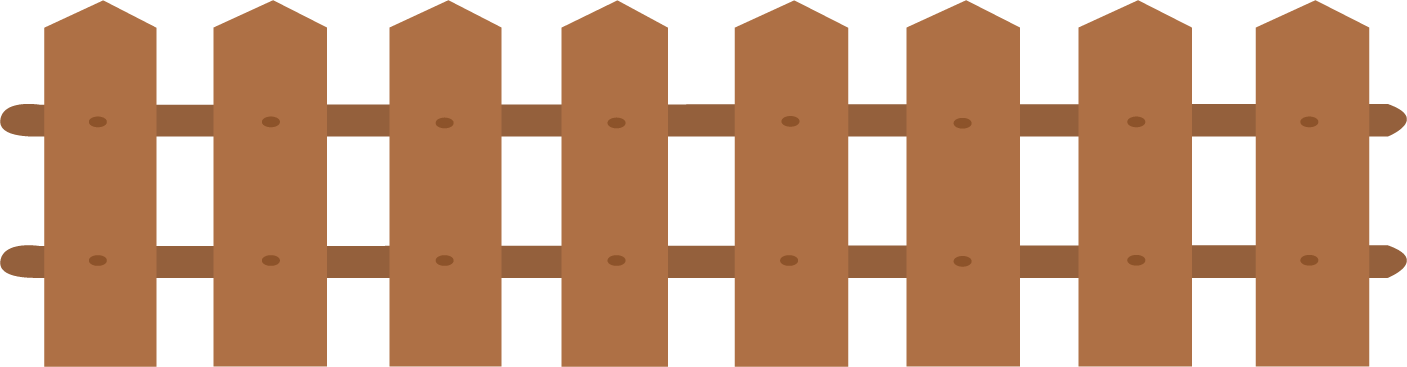Field and Fences

### Boundary

• The line around the outer edge of a shape is considered a boundary.

• The shape would be a perfect geometric shape or any irregular shape.

• The farms will not be regular always, the area will be unequal, boundaries will be uneven, etc.

### Measure of Boundary

• The finding of the length or size of something is measurement.

• Suppose if a farm is in a rectangle shape then take the length of all sides then by adding all these sides we get the boundary value.

• The Mathematical term ‘Perimeter’ can be used instead of a boundary.

Solved example 1: Boundary = ………… m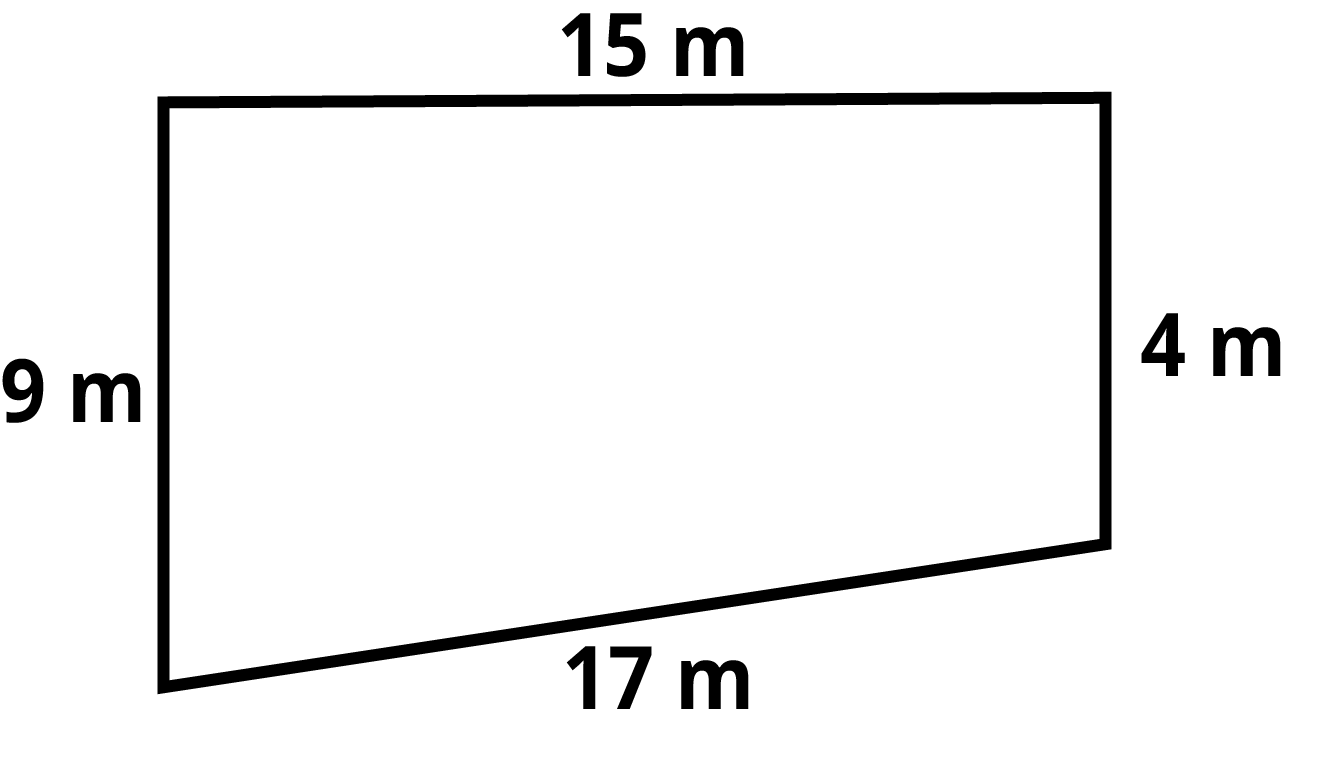Measure of boundary

Ans: We know that we get the total boundary value by adding up the length of all the sides, hence boundary value is,

15 + 9 + 4 + 17 = 45 m

Solved example 2: If the perimeter of a given image is 16cm, then the value that replaces the question mark is?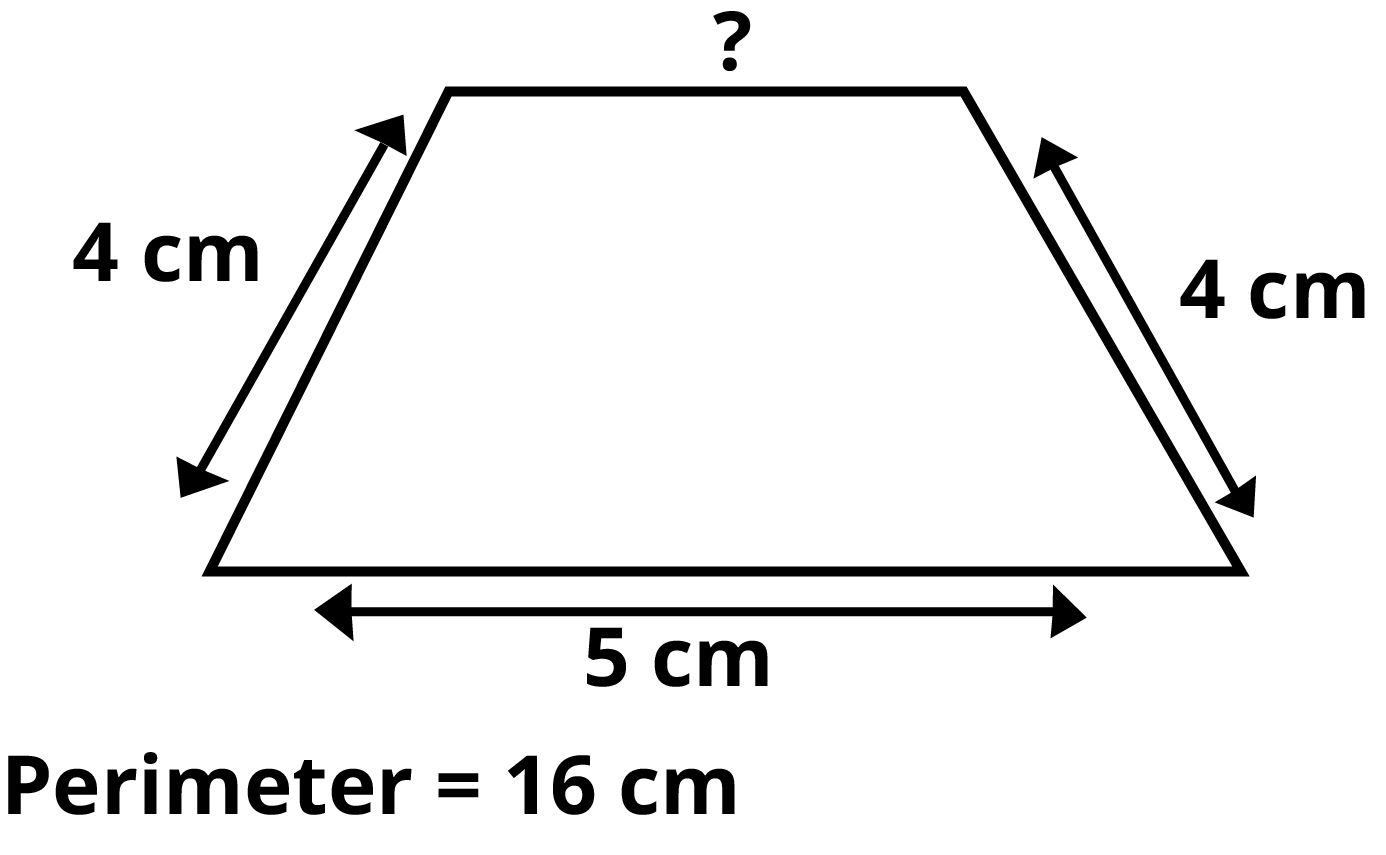Perimeter of the given figure

Ans: The sum of the lengths of all the sides of a given figure gives us a boundary or perimeter.

Here we have a perimeter, hence equating the sums to the perimeter we get,

4 + 4 + 5 + ? = 16 cm

= 16 - 13

= 3 cm

Solved example 3: Find out the perimeter of this football court.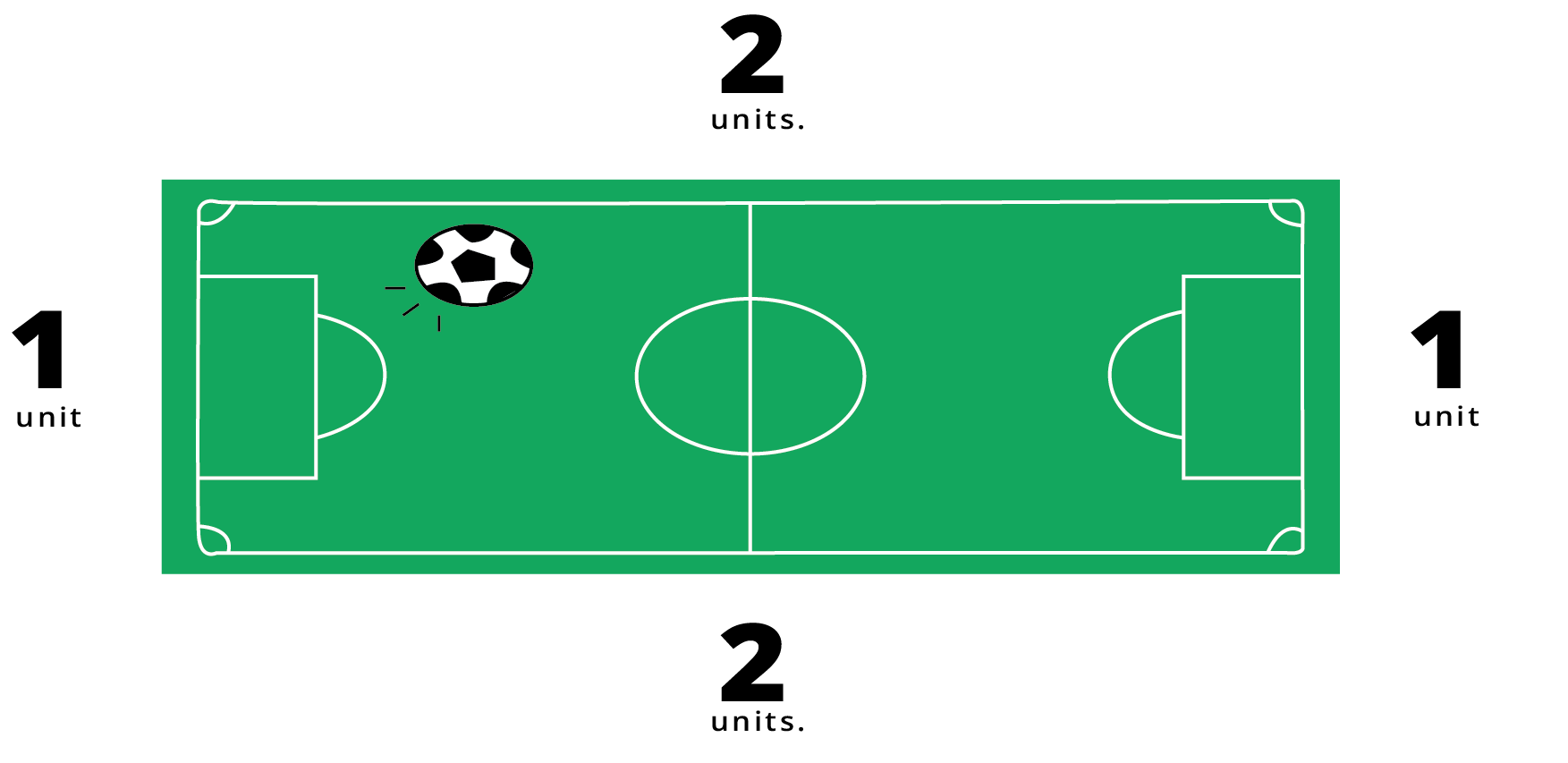Perimeter of football court

Ans: The boundary of a given figure can be obtained by adding all the sides of it. Hence

2 + 2 + 1 + 1 = 6 units

### The Boundary of Simple Shapes

• For simple shapes such as rectangles, squares, triangles, etc. we can find the perimeter easily.

• It can be done by just adding the measurement of the sides.

Solved example 1: Rina wants to put a table cloth for a table in her dining hall. Can you help her by measuring the perimeter of the table?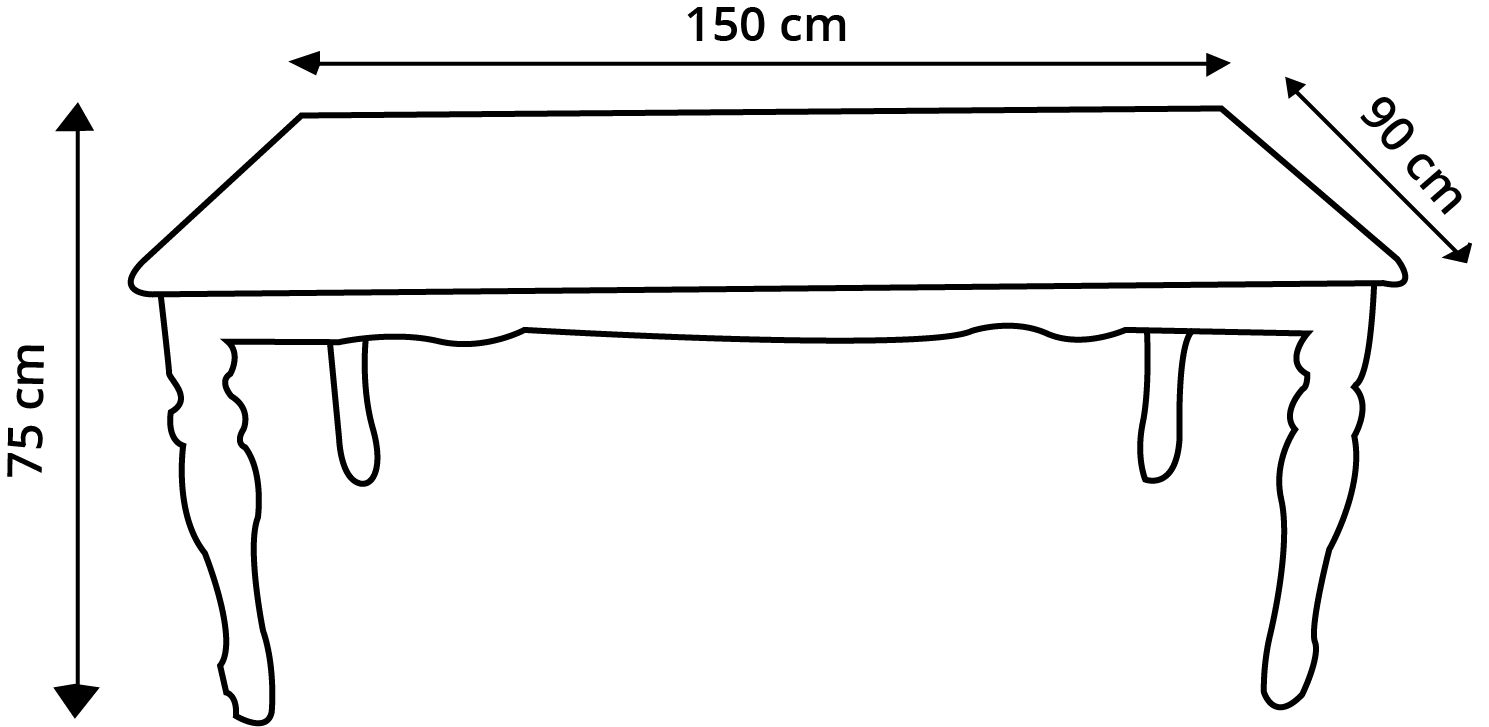Perimeter of table

Ans: Here Rina wants to put a table cloth on the table so the measurements are already given. If we add the length of the sides of a table we get the perimeter of the table.

Perimeter = 150 cm + 90 cm + 150 cm + 90 cm

Perimeter = 480 cm

Hence Rina can get a table of the perimeter of 480 cm.

Solved example 2: Shailu is planning to buy a carpet for her living room but she is confused about how to find the perimeter of the given place. In the image given below, find the perimeter of the space coloured in blue.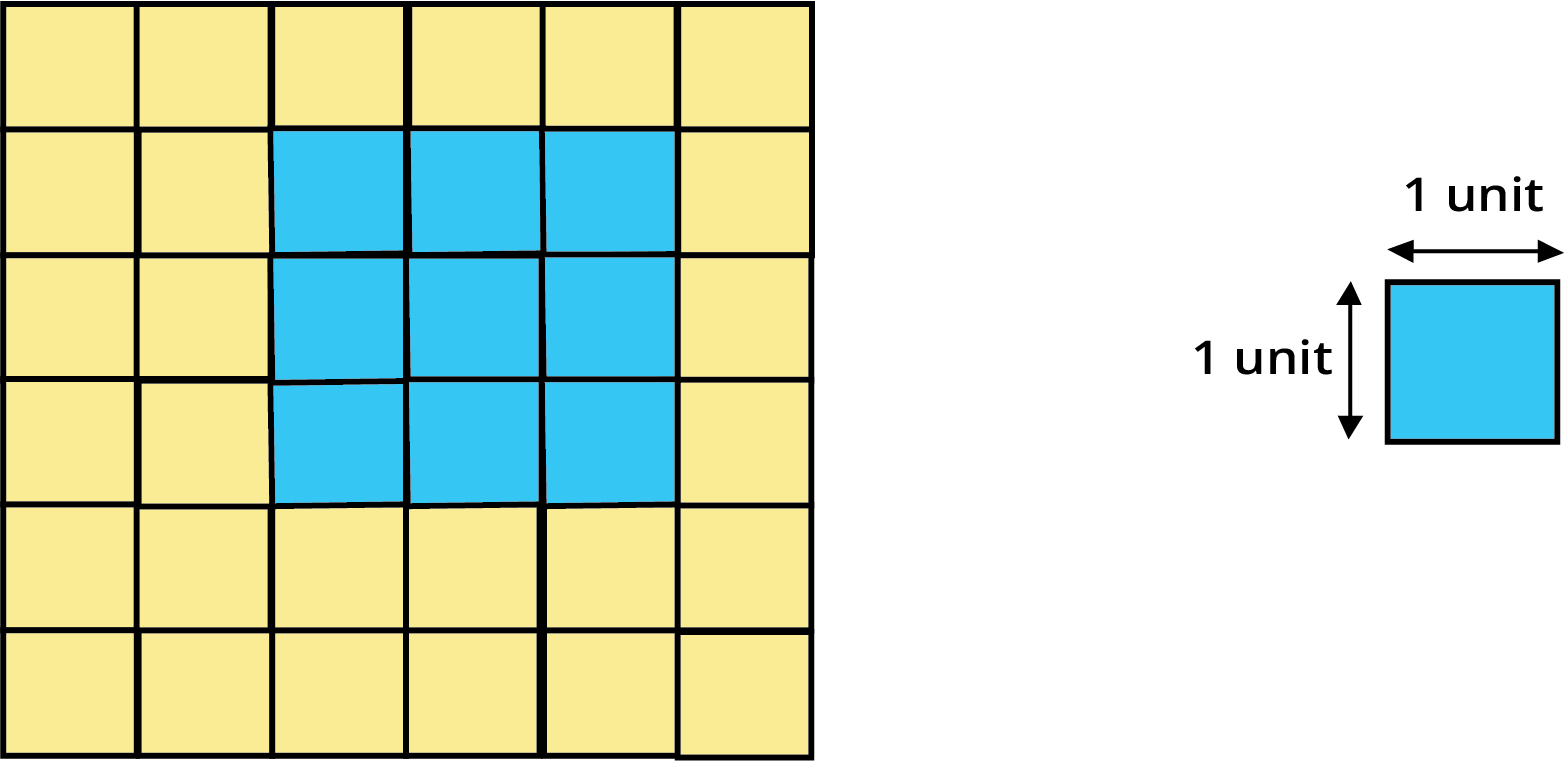Perimeter of the carpet

Ans:

In the image, it is shown that the blue-coloured space has 9 squares. Here for 1 square, the length is 1 unit on all sides.

Since on each side, we have 3 squares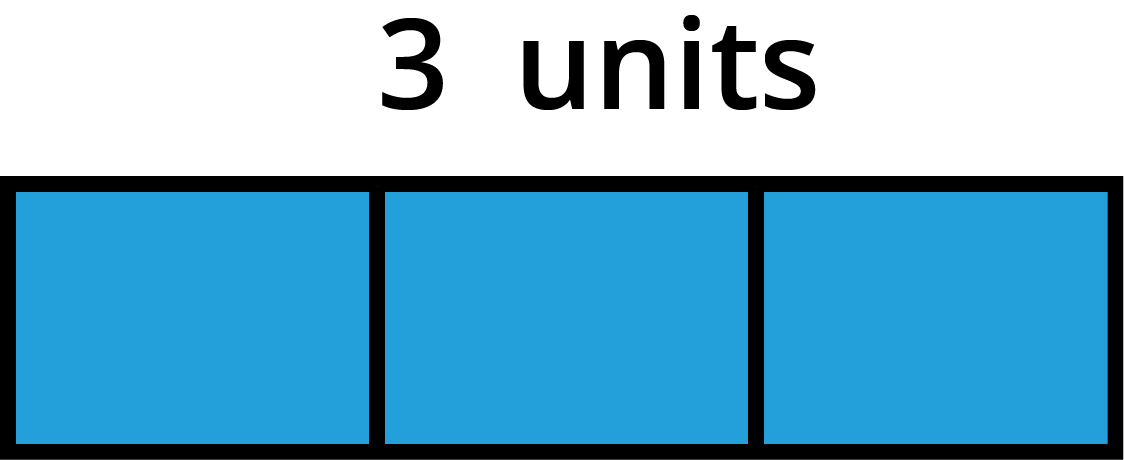We can apply this method to all sides.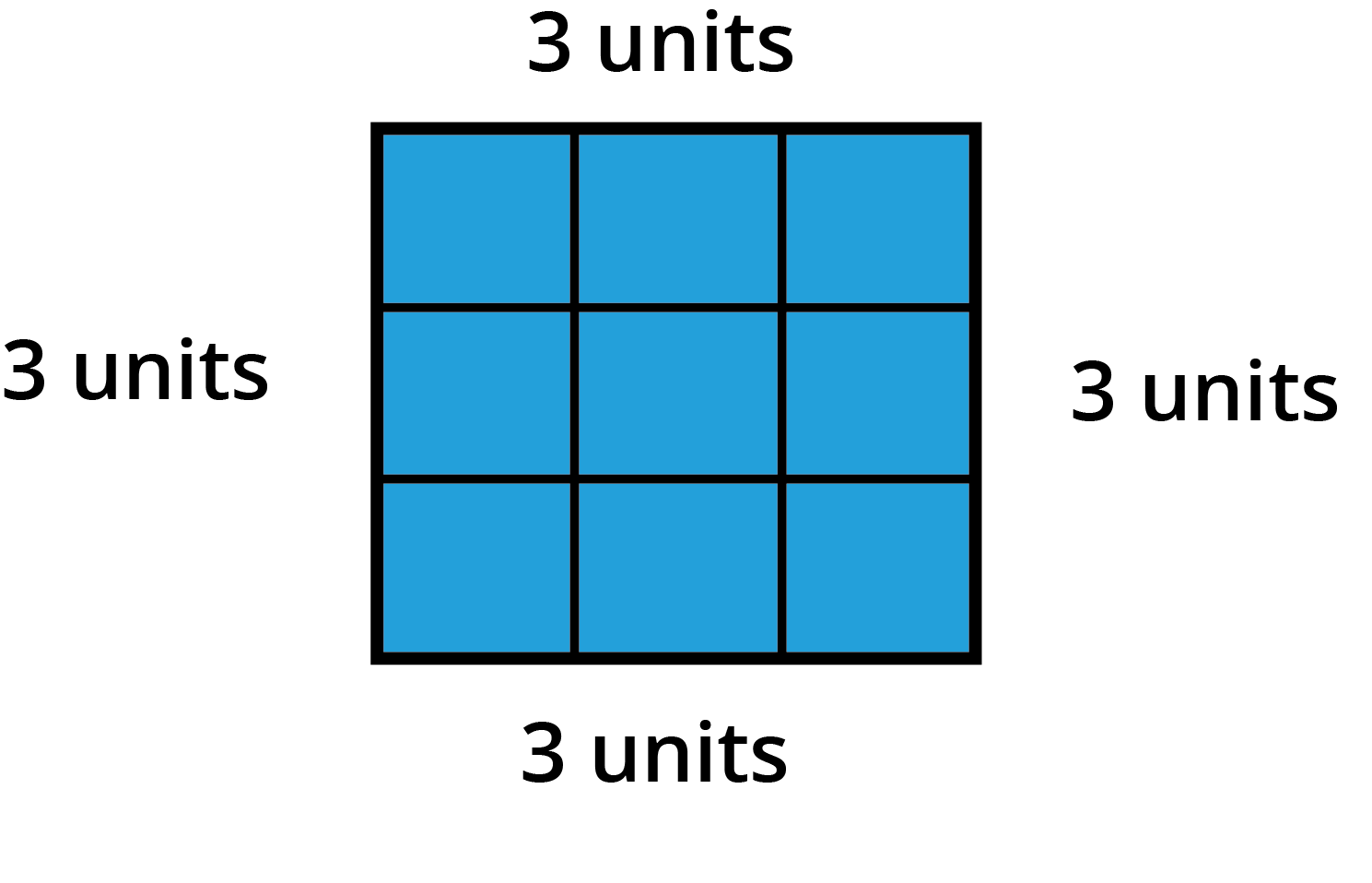Hence perimeter = 3 units + 3 units + 3 units + 3 units = 12 units

### Boundary of Complex Shapes

• We can easily solve the perimeter of the shapes such as rectangles, squares, etc.

• But if we want to measure the perimeter of some random shape. You can take the help of a thread.

• Take a thread and wrap it around its boundary then mark the place where it covers the boundary fully.

• Now measure the length using a scale.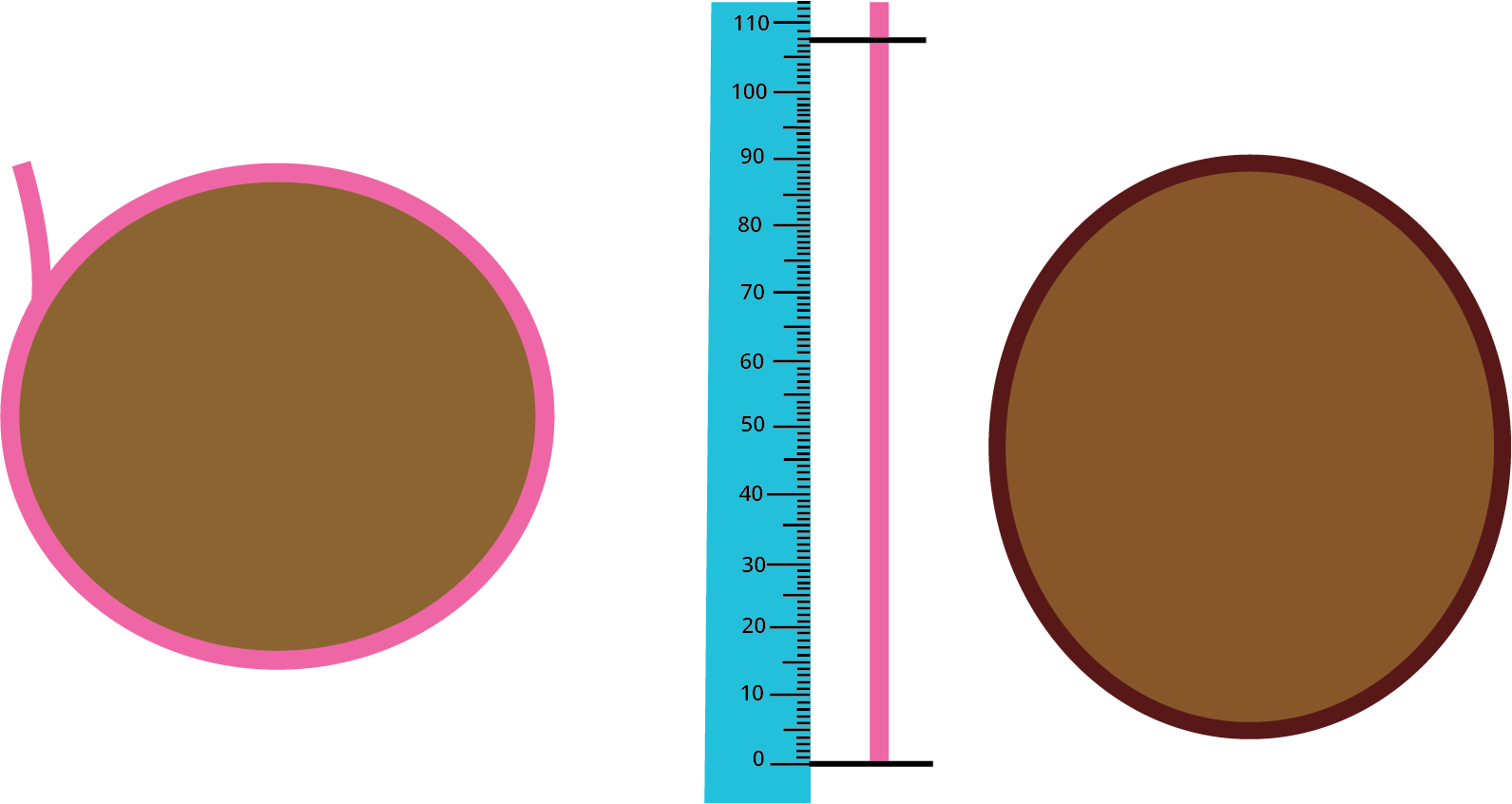Boundary of complex shapes

### Examples:

Solved example 1: Find the perimeter of the given shape.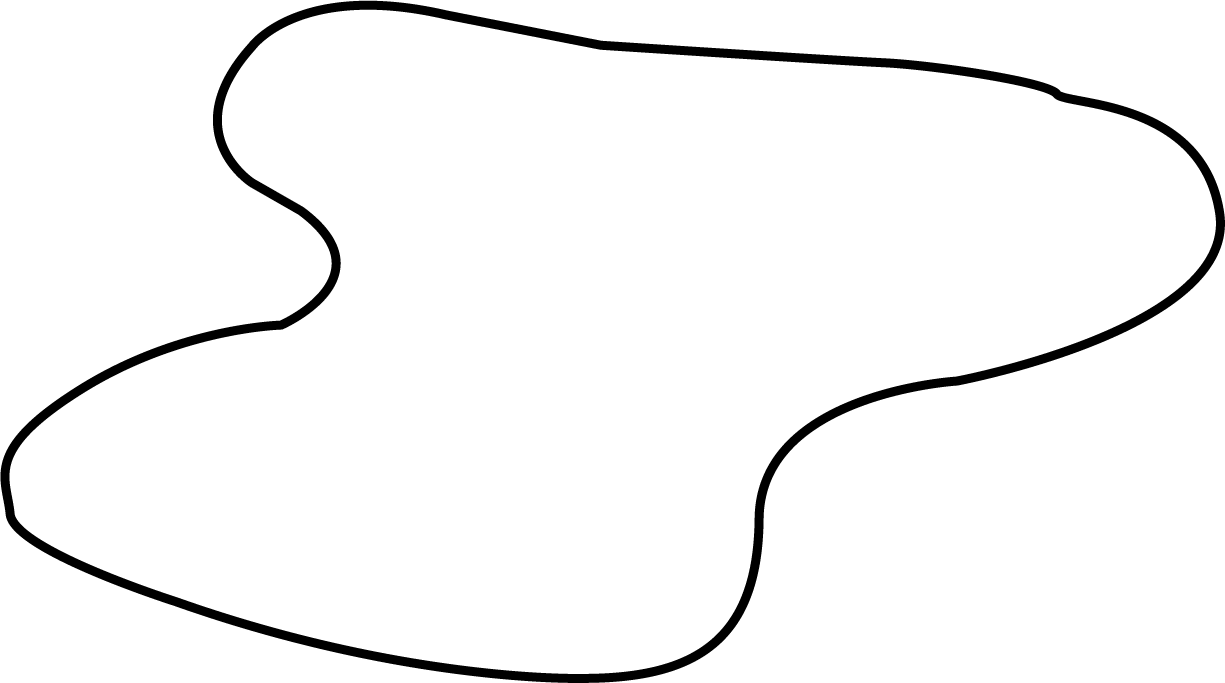Perimeter of the given shape

Ans: By following the steps given above, we can easily find the perimeter of the given shape.

• Firstly take a thread and wrap it around the edges.

• Now mark the place where it covers the boundary or the starting point.

• Now take a scale and measure the length of the thread till the marked point.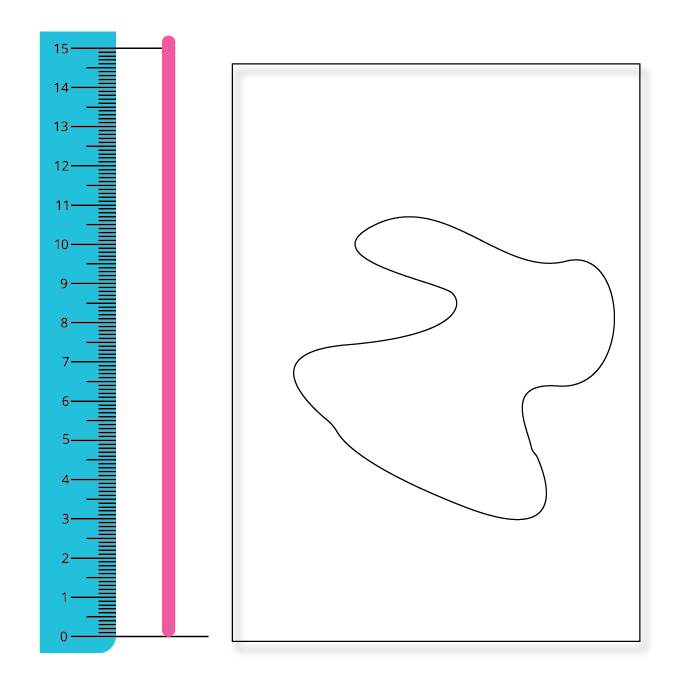Measurement of the perimeter with the help of scale

Hence the perimeter of the given shape is 15cm.

Solved example 2: In the given image you can put one plant in a complete square that is filled with greenery. Find the number of plants you can put in the given image.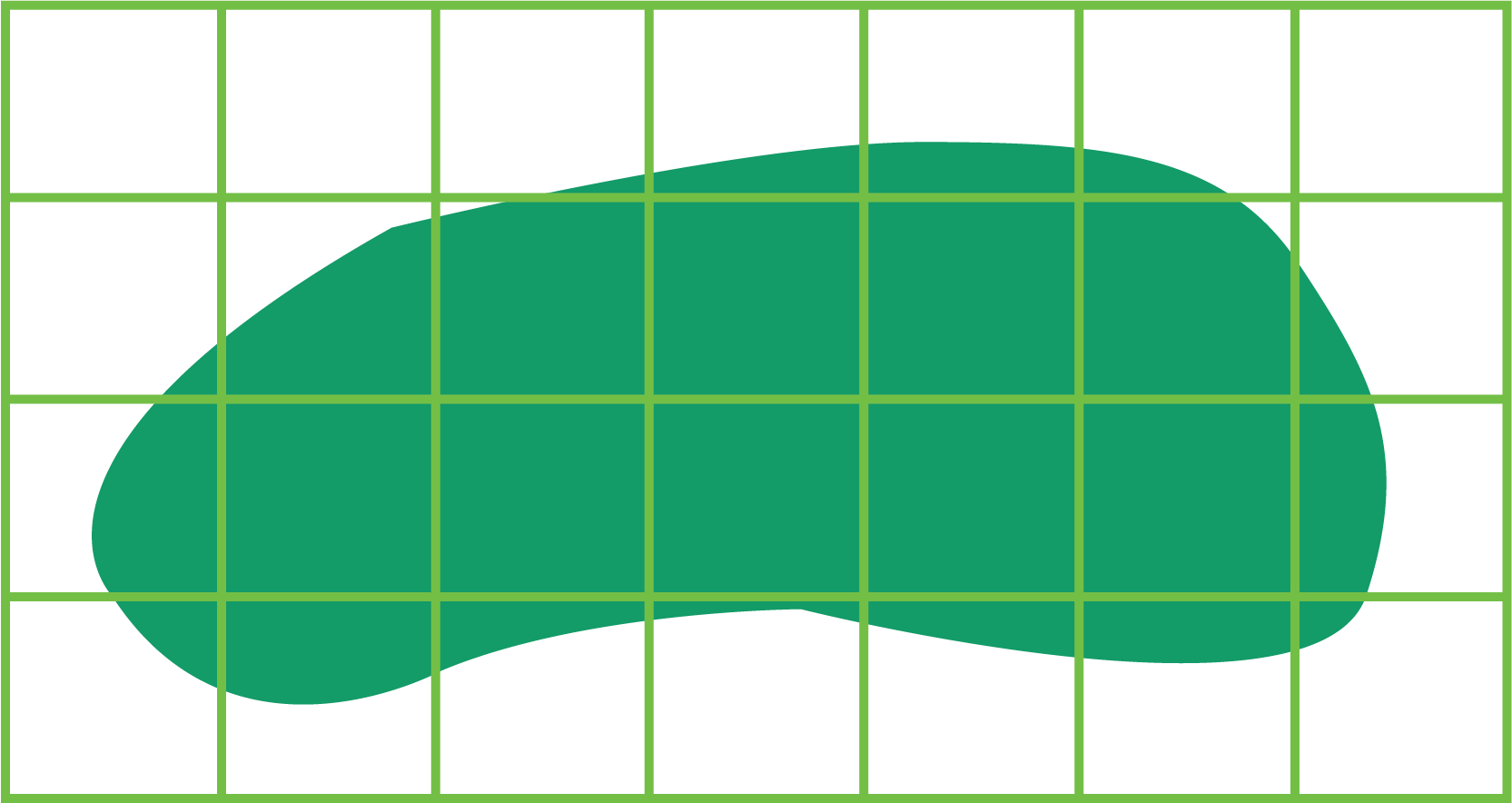Area of the green part

Ans: As per the question, to put a plant, we need a complete square that is filled with green colour. Hence the final answer will be as shown below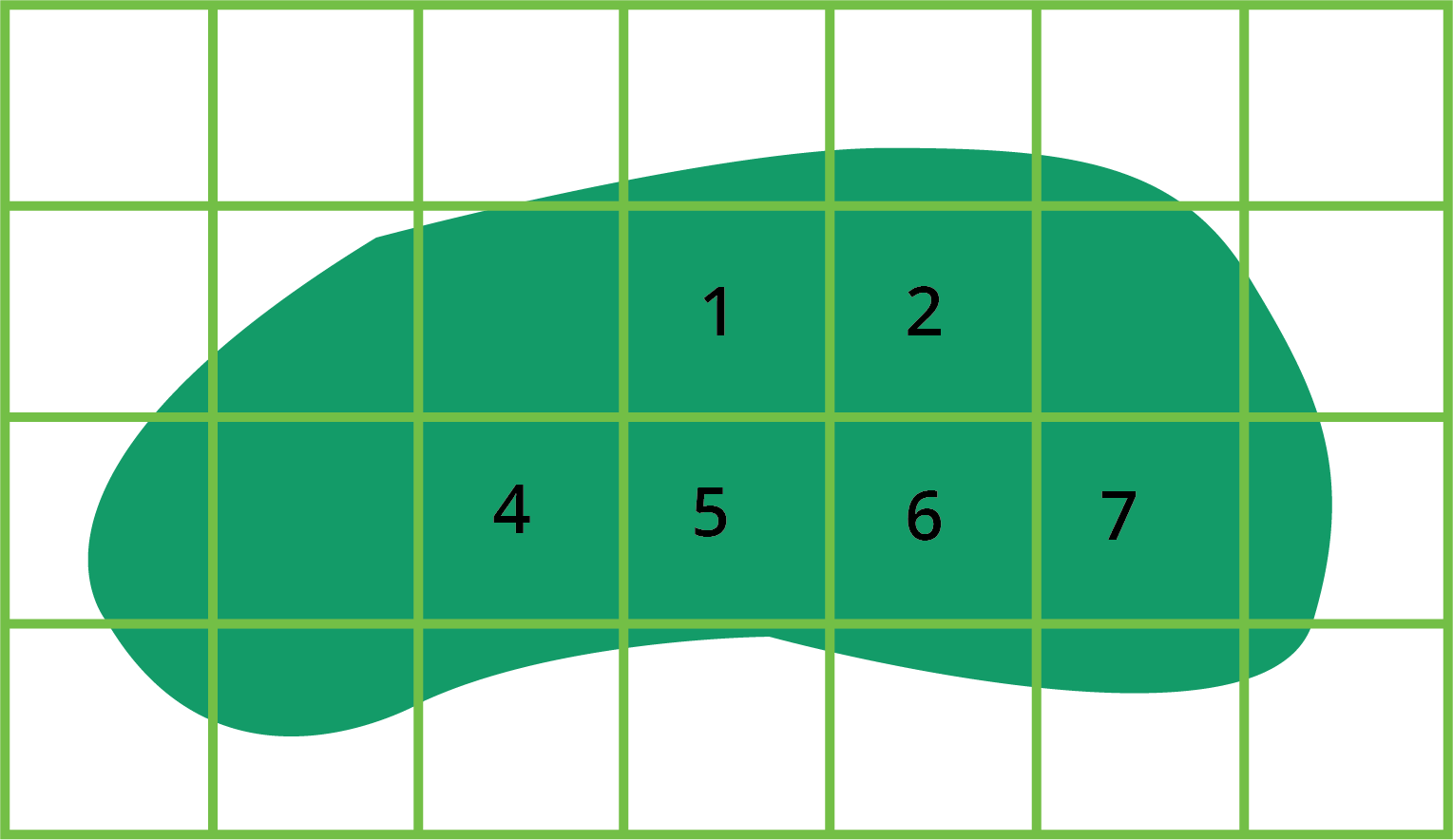Area of green part with the help of small squares

Solved example 3: Find the total number of squares required to completely fill the image given.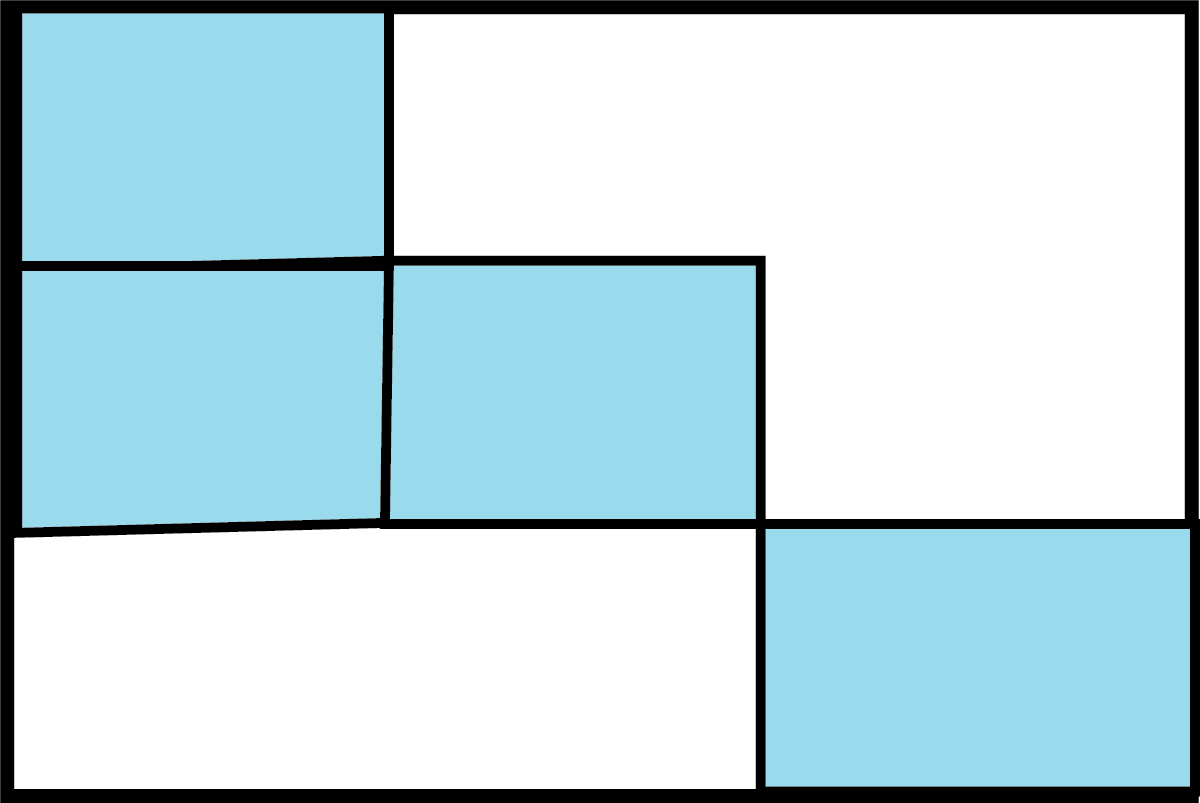A box

Ans:

• In the first line, we have one square, in the second line we have two squares, and in the third line, we have one square.

• Now if we observe carefully, we can extend each corner of the squares to the outer line with the help of a dotted line.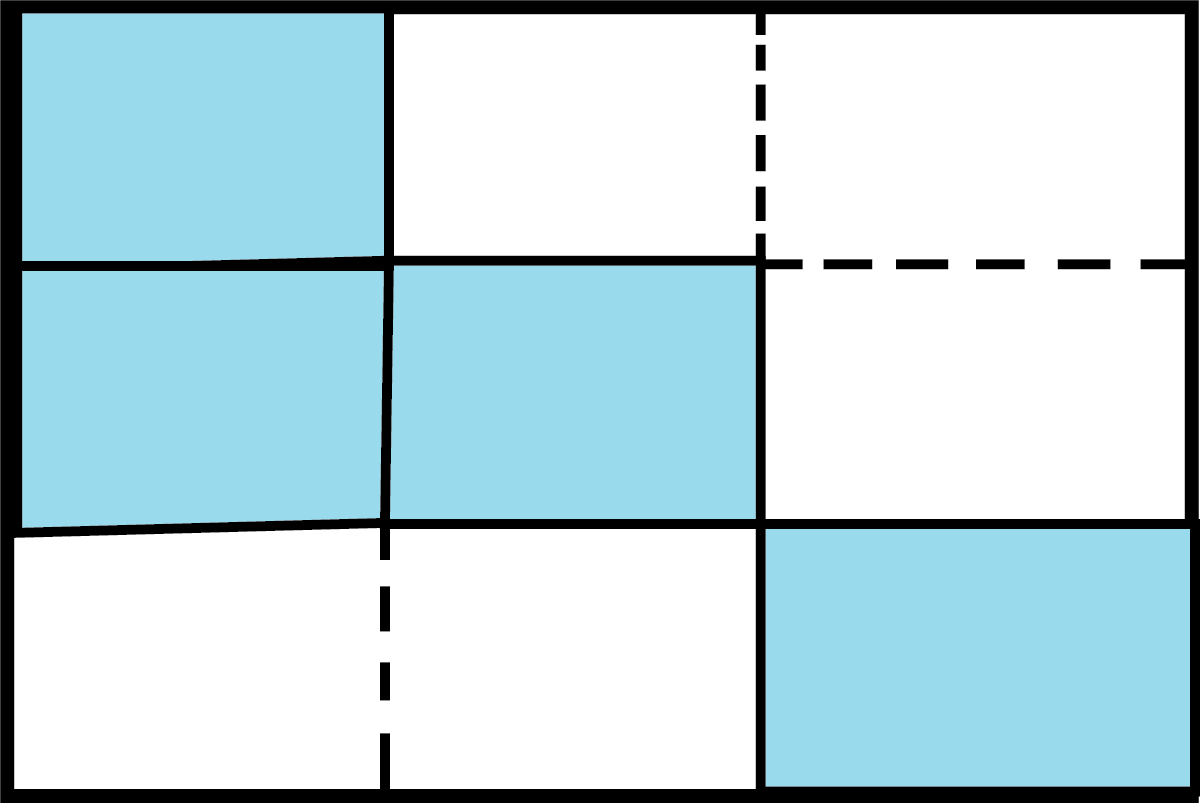• If we join those dotted lines we get the missing squares with matching lengths.

• The final image is shown below.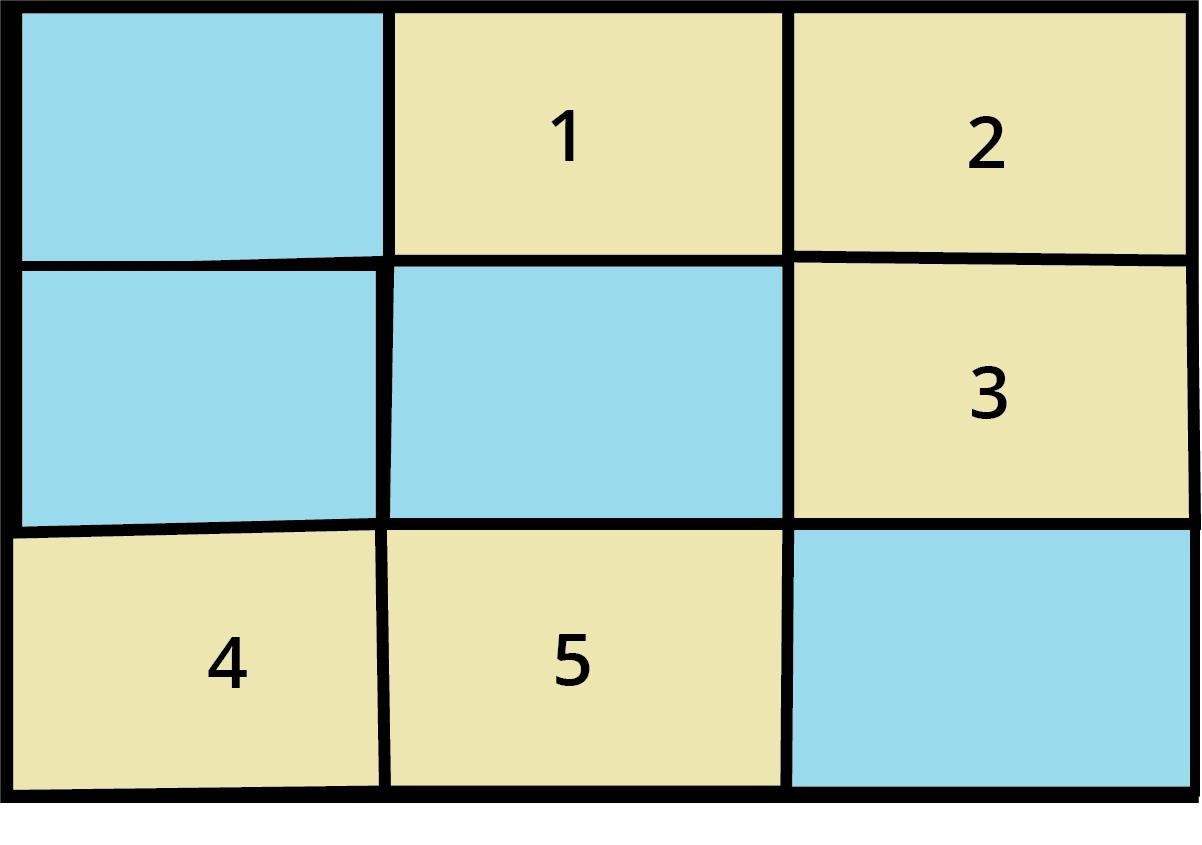### Practise Questions

1. Find the boundary length of the land shown in the image below.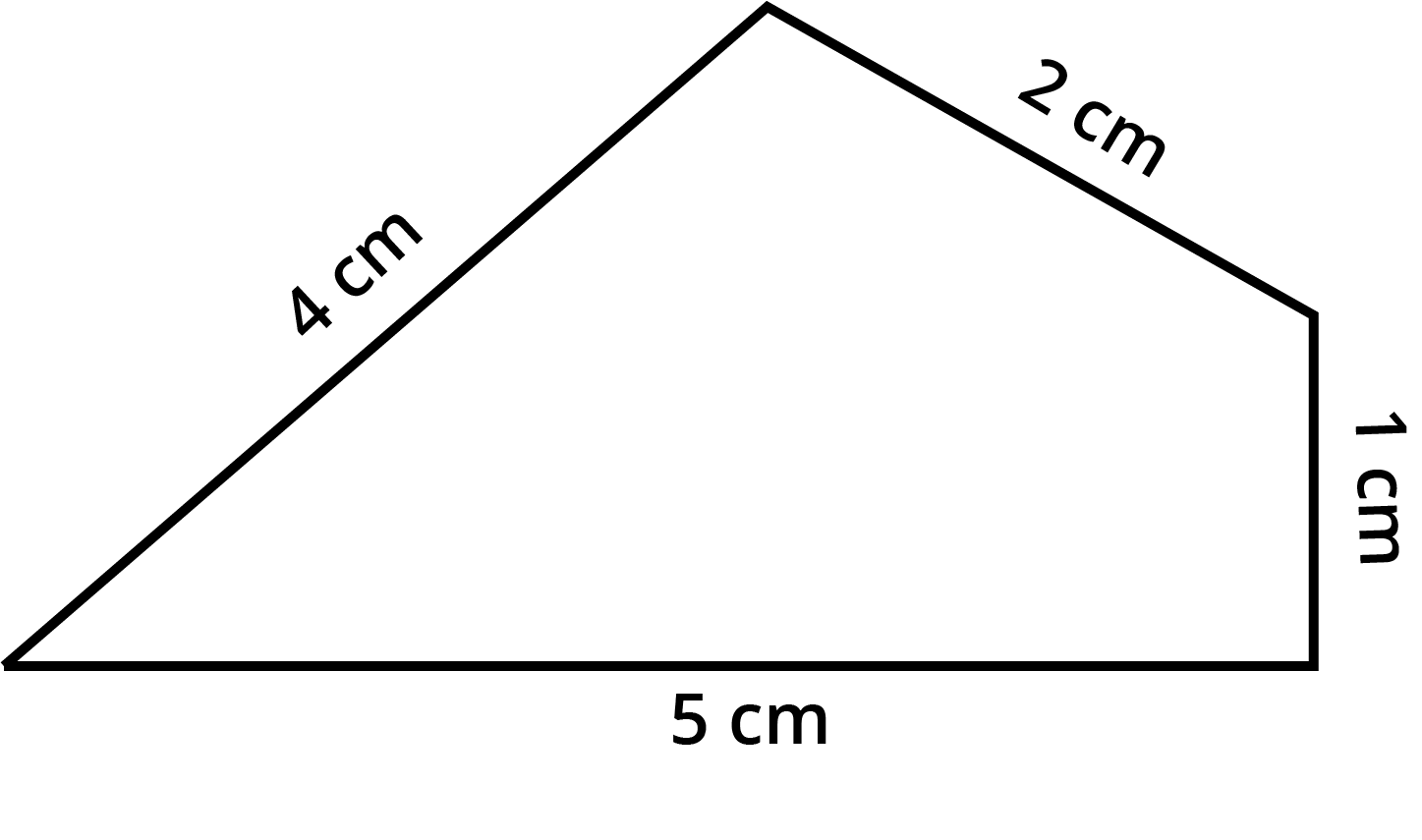(a) perimeter of a given shape

2. For the land that is shown in the image, find out the length of the wire required to fence its boundary.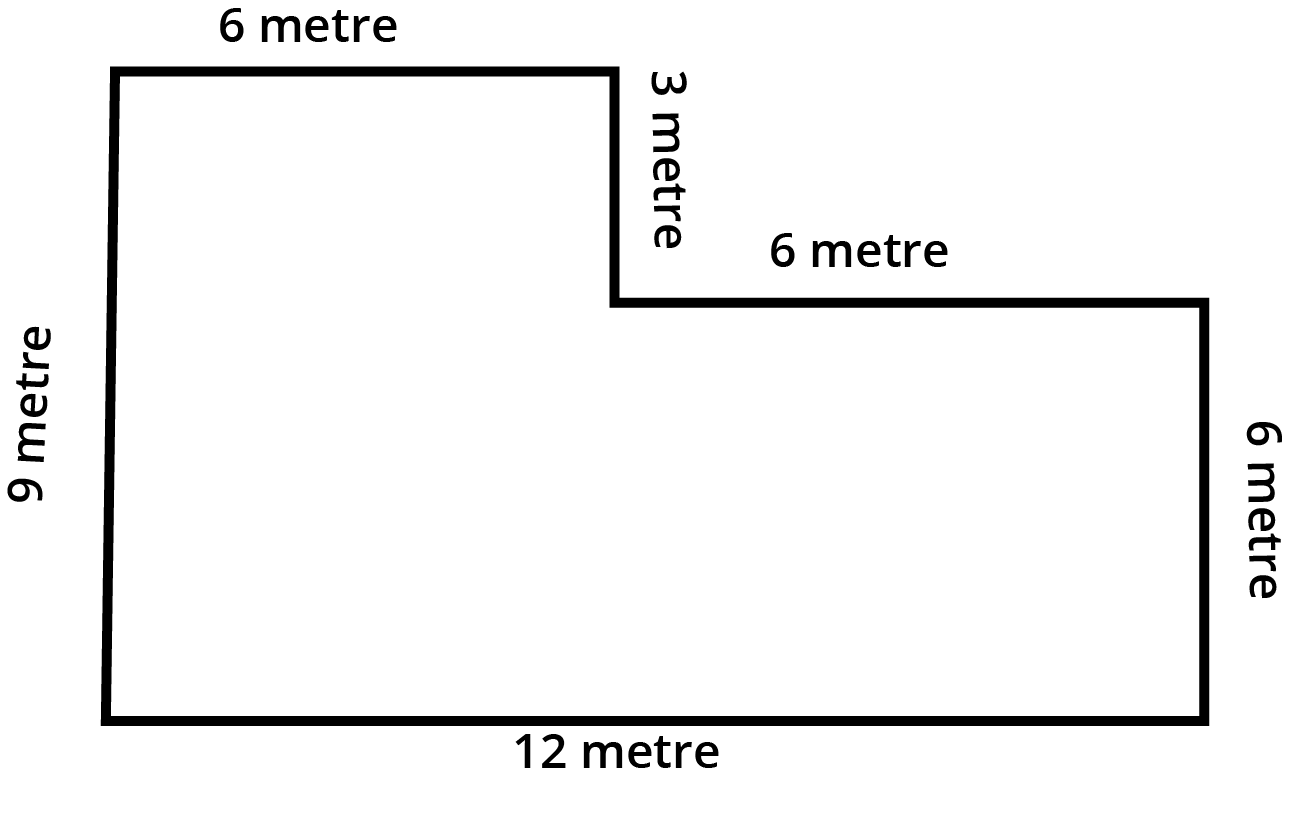(b) Perimeter of the given shape

## Importance of CBSE Class 4 Maths Chapter 13 Fields and Fences

All the field shapes in this chapter do not resemble the regular shapes that you might have studied in the previous classes. This chapter will explain what is field in mathematics and will teach you how to find out the length of a fence for all the field shapes.

The entire chapter has a proper explanation given using pictorial illustrations. Students will learn how to seek information given in the pictures and use it to solve the questions. The objective of this chapter is to develop a conceptual foundation of the perimeter of shapes among students by using simple examples of fields and fences. Students can also practice using units to define the perimeter of the length of a fence.

## Benefits of Field and Fences Class 4 Worksheets and Revision Notes

Our subject experts have prepared the revision notes and worksheets following the CBSE Class 4 guidelines. It will be easier for you to comprehend the concepts by referring to these revision notes as our experts have used simple language to prepare these notes.

All the shapes of fields are explained properly in these notes. You can correlate the solutions for Field and Fences Class 4 worksheets with the concepts. Learn how to use multiplication methods to calculate the length of a field’s boundary, provided the shape of the field is regular.

## Download Worksheets and Revision Notes for Class 4 Maths Chapter 13

Download the revision notes and worksheets of NCERT Class 4 Maths Chapter 13. You can refer to these revision notes once you have completely studied the chapter to revise the concepts quickly before the exam. Solve Class 4th Maths sums by using the NCERT Solutions to learn the standard answering patterns for these sums.

It will be easier to understand the concepts and objectives of this chapter from the Field and Fences summary. Follow the NCERT Solutions prepared by our experts for the worksheets to get a better understanding of the concepts and enhance your skills.

## FAQs on Fields and Fences Class 4 Notes CBSE Maths Chapter 13 (Free PDF Download)

1.  How will the Class 4th Maths Chapter 13 NCERT Solutions help me?

All the answers to the questions in the exercise and worksheets given here will help you understand the question patterns and easy solutions to the questions. You will be able to recognize the pattern and answer them accordingly by recalling the solution methods given by our experts.

2.  Why is the Chapter 13 Math activity for Class 4 important?

The activities are designed to deliver a hands-on experience to the students. This is an excellent method of experiential learning that helps you to understand what perimeter is and how to calculate it.

3.  How can I measure the length of the boundary of a field?

If the field is irregular in shape, use a rope. If it is a rectangle, measuring its length and breadth will do the trick. If it is a square, you can multiply its side’s length by 4. If it does not have a proper shape but has sides, measure all the sides and add them to get an answer.